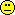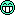BEQool
☆

Slovenia,
2023-05-24 23:05
(128 d 06:06 ago)

Posting: # 23567
Views: 933

## Sample size estimation for parallel design with more than 2 treatments [Power / Sample Size]

Hello all!

I have a question regarding sample size estimation for parallel designs with more than 2 treatment arms (with more than 2 groups). Package PowerTOST in R covers sample size estimation for parallel design for just 2 parallel groups (known.designs()) and not for more.
Lets say we are conducting a (pilot) parallel study with 3 test formulations and 1 reference. How do we estimate sample size in this case?

I would say that we just double the necessary sample size estimated (in R with sampleN.TOST) for 2 parallel groups (to get 4 independent groups for 3 test formulations and 1 reference). For example for CV=0.2 (parallel design and theta0=0.95) for 2 parallel groups we get sample size of 36 subjects (total sample size for 2 groups), so if we double it we get 72 subjects (for 4 groups?). Is this assumption and sample size estimation a correct one?

On the other hand I have read this article regarding multiple treatments comparison in parallel design and I dont exactly understand why should we just control Type I Error and change alpha when estimating sample size with multiple treatments? So in our case (when using Bon­ferroni adjustment) we would get the total sample size (for 4 groups) of 50 subjects (parallel design, CV=0.2, theta0=0.95, alpha=0.0167)? Is this correct? What is more, 50 is not even dividable by 4.I am confused and I probably didnt get this explanation in the article right. Nevertheless I wouldnt mind this being the right way as the needed sample size is smallerRegarding alpha adjustment in the first case (for doubling sample size from 36 to 72) I would say that it is not necessary as it is a pilot study. On the other hand, if this was a pivotal study and we would want to show equivalence for just 1 test formulation (among 3) then we would have to adjust alpha (to 0.0167) and then I would say we would double the estimated sample size for 2 groups the same way as described above (so 50x2=100 subjects)?

So which sample size estimation is a correct one?

BEQool
dshah
★★

India/United Kingdom,
2023-05-25 11:42
(127 d 17:29 ago)

@ BEQool
Posting: # 23568
Views: 753

## Sample size estimation for parallel design with more than 2 treatments

Hello BEQool!

When we refer to EMA BE guideline, the BE estimation shall be considered for the concerned treatment and the rest of the treatment shall be separated from calculation purpose.

So if there are more than two treatments, why can't the sample size be considered separately and then take a overall decision?
Consider below case.

Case 1:
Desired Power 80%
r (note: nT*r=nR) 1
a 5%
Total CV 40.00%
Expected mT/mR Ratio 95.00%
Lower Equivalence Limit 80.0%
Upper Equivalence Limit 125.0%

Required Sample Size 65 (nA)+65 (nB)=130 (Total)

Case 2:
Desired Power 80%
r (note: nT*r=nR) 1
a 5%
Total CV 40.00%
Expected mT/mR Ratio 90.00%
Lower Equivalence Limit 80.0%
Upper Equivalence Limit 125.0%
1-b 0.800076232

Required Sample Size 133 (nA)+133 (nB)=266 (Total)

Desired Power 80%
r (note: nT*r=nR) 1
a 5%
Total CV 20.00%
Expected mT/mR Ratio 95.00%
Lower Equivalence Limit 80.0%
Upper Equivalence Limit 125.0%

Required Sample Size 18 (nA)+18 (nB)=36 (Total)

And for 4 arm--> sample size is 72.

But why the assumption are same? what is the purpose of additional arm, if the expected ratio is same?

Regards,
Divyen
Helmut
★★★Vienna, Austria,
2023-05-27 10:26
(125 d 18:45 ago)

@ BEQool
Posting: # 23573
Views: 712

## >2 treatments, multiplicity or not

Hi BEQool,

❝ I have a question regarding sample size estimation for parallel designs with more than 2 treatment arms (with more than 2 groups). Package PowerTOST in R covers sample size estimation for parallel design for just 2 parallel groups (known.designs()) and not for more.

Correct, since one comparison is assumed.
sampleN.TOST(..., design = "parallel") – like all functions of PowerTOST – gives the total sample size.

❝ Lets say we are conducting a (pilot) parallel study with 3 test formulations and 1 reference. […] I would say that we just double the necessary sample size estimated (in R with sampleN.TOST) for 2 parallel groups (to get 4 independent groups for 3 test formulations and 1 reference). For example for CV=0.2 (parallel design and theta0=0.95) for 2 parallel groups we get sample size of 36 subjects (total sample size for 2 groups), so if we double it we get 72 subjects (for 4 groups?). Is this assumption and sample size estimation a correct one?

First get the number of subjects per group from the total sample size. Then multiply by the number of comparisons + 1. See the script at the end.

Tests                   : 3 Groups                  : 4 alpha                   : 0.05 Sample size per group   : 18 Total sample size       : 72 Achieved power          : 0.80994

❝ […] I have read this article regarding multiple treatments comparison in parallel design and I dont exactly understand why should we just control Type I Error and change alpha when estimating sample size with multiple treatments?

I agree that this section was poorly written.I revised it (press to refresh the browser cache).
In a pilot study we don’t have to adjust $$\alpha$$. Actually the CI is not relevant at all and therefore, we can use any (not too small) sample size. We are interested in the T/R-ratios and CVs for selecting the ‘best’ candidate (see case 1 in this article about higher-order Xovers) and planning the pivotal study.

❝ So in our case (when using Bon­ferroni adjustment) we would get the total sample size (for 4 groups) of 50 subjects (parallel design, CV=0.2, theta0=0.95, alpha=0.0167)? Is this correct?

Nope. Try the script with adj <- TRUE (potentially applicable in a pivotal study; see the explanations at the end):

Tests                   : 3 Groups                  : 4 alpha                   : 0.05 Multiplicity adjustment : Bonferroni Adjusted alpha          : 0.0166667 (alpha / 3) Sample size per group   : 25 Total sample size       : 100 Achieved power          : 0.80304

❝ What is more, …

Of course, $$\small{\alpha \downarrow\:\Rightarrow\:n \uparrow}$$

❝ … 50 is not even dividable by 4.But 100 is.❝ I am confused and I probably didnt get this explanation in the article right. Nevertheless I wouldnt mind this being the right way as the needed sample size is smallerSee above.

❝ Regarding alpha adjustment in the first case (for doubling sample size from 36 to 72) I would say that it is not necessary as it is a pilot study.

Correct.

❝ On the other hand, if this was a pivotal study and we would want to show equivalence for just 1 test formulation (among 3) then we would have to adjust alpha (to 0.0167) and then I would say we would double the estimated sample size for 2 groups the same way as described above (so 50x2=100 subjects)?

Correct. See also the ICH M13A draft guideline, Section 2.2.5.2 Multiple Test Products:
1. If all tests should pass (AND-condition), you don’t have to adjust $$\alpha$$.
2. If any of the $$k$$ tests should pass (OR-condition), you could use Bonferroni’s $$\alpha / k$$, which is the most conservative method.
As an aside, the GL states for the second case “multiplicity adjustment may be needed”. This allows for more complex and less restrictive adjustments, e.g., Holm-Bonferroni, Hochberg-Holm, hierarchical testing. Since we don’t know beforehand which test will perform ‘best’, estimate the sample size for Bonferroni, gaining power for the others.
Furthermore, we don’t need an adjustment when comparing one test to comparators from two regions.

library(PowerTOST) alpha0 <- 0.05  # nominal (overall) level CV     <- 0.2   # assumed total CV theta0 <- 0.95  # assumed T/R-ratio(s) target <- 0.8   # target (desired) power k      <- 3     # number of tests adj    <- FALSE # FALSE in a pilot study and in a pivotal study if all tests should pass                 # TRUE in a pivotal study if any of the tests should pass if (!adj) {     # unadjusted   alpha <- alpha0 } else {        # Bonferroni   alpha <- alpha0 / k } # Total sample size and power for two groups tmp    <- sampleN.TOST(alpha = alpha, CV = CV, theta0 = theta0, targetpower = target,                        design = "parallel", print = FALSE) N      <- tmp[["Sample size"]] pwr    <- tmp[["Achieved power"]] # Total sample size for k (tests) + 1 (reference) groups n      <-  N * (k + 1) / 2 txt    <- paste0("\nTests                   : ", k,                  "\nGroups                  : ", k + 1) if (adj) {   txt <- paste0(txt, "\nalpha                   : ", alpha0,                      "\nMultiplicity adjustment : Bonferroni",                      "\nAdjusted alpha          : ", signif(alpha, 6),                      " (alpha / ", k, ")") } else {   txt <- paste0(txt, "\nalpha                   : ", alpha0) } txt    <- paste0(txt, "\nSample size per group   : ", N / 2,                       "\nTotal sample size       : ", n,                       "\nAchieved power          : ", signif(pwr, 5), "\n") cat(txt)

Dif-tor heh smusma 🖖🏼 Довге життя Україна!Helmut SchützThe quality of responses received is directly proportional to the quality of the question asked. 🚮
Science Quotes
BEQool
☆

Slovenia,
2023-05-29 12:41
(123 d 16:30 ago)

@ Helmut
Posting: # 23576
Views: 613

## >2 treatments, multiplicity or not

Thank you Divyen and Helmut, everything is clear and resolved now.

❝ I agree that this section was poorly written.I revised it (press to refresh the browser cache).

It was surely written well enough (just like other articles), it was probably just me who didnt exactly get itBy the way, thank you for these articles, they are excellent!

BEQoolIng. Helmut Schütz